# Create your own formulas: use the correct JavaScript syntax for each type of calculated property

Calculated properties are custom properties whose values are calculated from a formula. The formula can include the values of other properties, thereby creating dependencies between your properties. Library administrators can create calculated properties for all property types and in all types of library.

This article explains the JavaScript syntax you can use in each type of calculated property. It also provides examples of the corresponding calculated values you can expect.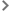Note about different types of JavaScript syntaxCalculated String or Text propertiesCalculated Integer propertiesCalculated Decimal propertiesCalculated Boolean propertiesCalculated Date, Date&Time or Time propertiesCalculated Category properties

## Note about different types of JavaScript syntax

In the examples we give in our articles about calculated properties (learn more: Getting started with formulas for calculated properties), we use two types of JavaScript syntax:

• single expression
• multiple instructions, wrapped in an arrow function

You can use either in your calculated properties, but we recommended taking account of the following considerations.

General rule: the JavaScript code defining a formula must be made of one, and only one, expression.

This means that:

• if your logic can be expressed in a single expression, you can type it directly in the formula builder, for example:

`property`Project name`+' - '+sequenceId`document``

• if your code requires additional instructions (such as when processing dates), you should wrap all your statements in an arrow function definition `(() => { ... })`, and call it right after the definition with an arrow function call `()`:

``` (() => { const d=new Date(property`Publication date`) const yyyy=d.getFullYear(), const mm=`\${d.getMonth()+1}`. padStart(2,'0') const dd=`\${d.getDate()}`.padStart( 2,'0') return `\${mm}/\${dd}/\${yyyy}` })() ```

However, you can play with JavaScript to combine these multiple instructions into a single expression on a single line:

``` `\${`\${(new Date(property`Publication date`)) .getMonth()+1}`. padStart(2,'0') }/\${`\${(new Date(property`Publication date`)) .getDate()}`.padStart( 2,'0') }/\${(new Date(property`Publication date`)).getFullYear()}`  ```

You can then enter this directly in the formula builder without using the arrow function definition.

We recommend using the arrow function definition because with the single-line expression:

• there's a lot of repetition, which is not good practice; in our example ```new Date(property`Publication date`)``` is repeated three times
• the expression is long, which makes it difficult to read and debug

## Calculated String or Text properties

String and Text properties accept as input:

• Strings
• Integers
• Decimals
• Booleans

They also support simple math calculations, which are evaluated when the formula is calculated and the result of which is displayed. Learn more: Use simple math calculations.

Below are some supported JavaScript expressions with their expected values.

 JavaScript expression JavaScript type Value of calculated property Comment `'Document validated'` StringValue: `Document validated` Single quotes `"Document validated"` String Value: `Document validated` Double quotes `1234` Number Value: `1234` No formatting `3.0` Number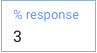Value: `3` No formatting `1+2` Number Value: `3` Evaluation first `true` or`false` Boolean Value: `true` or`false` English ``\${3+4} documents`` String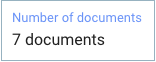Value: `7 documents` String literal

## Calculated Integer properties

The nominal expected value is a JavaScript Number, but a best effort is made to also map String types.

Below are some supported JavaScript expressions with their expected integer values.

 JavaScript expression JavaScript type Value of calculated property Comment `42` Number Value: `42` Nominal integer `1+2` Number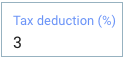Value: `3` Operation `5.0` Number Value: `5` No decimal part `3.14` Number Value: `3` Round-down `3.8` Number Value: `3` Round-down `'12'` String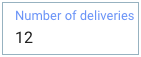Value: `12` String parsing integer `"8.2"` String Value: `8` String parsing decimal

## Calculated Decimal properties

The nominal expected value is a JavaScript Number, but a best effort is made to also map String types.

Below are some supported JavaScript expressions with their expected decimal values.

 JavaScript expression JavaScript type Value of calculated property Comment 3.14 Number Value: `3.14 ` Nominal decimal 1.2+3.8 Number Value: 5 Operation 4 Number Value: 4 Integer 2 + 3.1 Number Value: `5.1 ` Hybrid operation ‘6.626’ String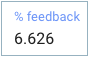Value: `6.626` String parsing decimal 1 + 0.61 Number Value: `1.61 `(not 1.6099999999999999) Double precision:16 bits

## Calculated Boolean properties

The nominal expected value is a JavaScript Boolean, but a best effort is made to also map String types.

Below are some supported JavaScript expressions with their expected boolean values.

 JavaScript expression JavaScript type Value of calculated property Comment `true` or `false` BooleanValue:`true` or `false`(in this example, `true`) Nominal `‘true’` or `‘false’` StringValue:`true` or `false`(in this example, `false`) String in english `‘TRUE’` or `‘FALSE’` String Value: `true` or `false` String in ENGLISH

## Calculated Date, Date&Time or Time properties

The nominal expected values are either JavaScript Date or Number. Strings are not supported.

Notes:
– Dates and times are given in Epoch milliseconds (the number of milliseconds since January 1, 1970, 00:00:00 UTC).
– Months in JavaScript start at zero: January is 0, February is 1, and so on. Learn more: Extract the month.
–  Date properties must use a value that corresponds to midnight UTC, otherwise an error occurs.
– The display of dates varies according to the user's locale. For example, en_GB, en_US and fr_FR all display dates differently.

Below are some supported JavaScript expressions with their expected Date values.

 JavaScript expression JavaScript type Value of calculated property Comment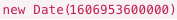Date AODocs Date property:JavaScript Date `483211100000` Number AODocs Date&Time property: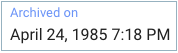Value: `April 24, 1985 7:18 PM` AODocs translates the number into a JavaScript Date `1607544327000` Number AODocs Time property: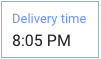Value: `8:05 PM` AODocs translates the number into a JavaScript Date `new Date(2015,3,1)` Date AODocs Date&Time property: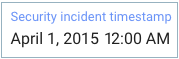Value: `April 1, 2015 12:00 AM` Note: If you don't indicate time in the code, the time defaults to 12 AM UTC (or 12 AM UTC plus however many hours ahead of UTC your timezone is). JavaScript Date `new Date(2015,3,1,10,30)` Date AODocs Date&Time property: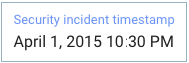Value: `April 1, 2015 10:30 PM` JavaScript Date

## Calculated Category properties

The nominal expected value is a String equal to the selected category value ID

 JavaScript expression Structure of the category value entity reference:#{categoryValue:category_ID/category_value_ID} For example: `'#{categoryValue:SGb0BhbtJUsWGRDo3z/SGb2pVav2f0yuuwpp9}'` Important: Use the code injector in the Formula pop-up. Learn more: Create calculated properties. JavaScript type String Value of calculated Category property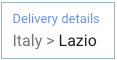Value: `Italy > Lazio`